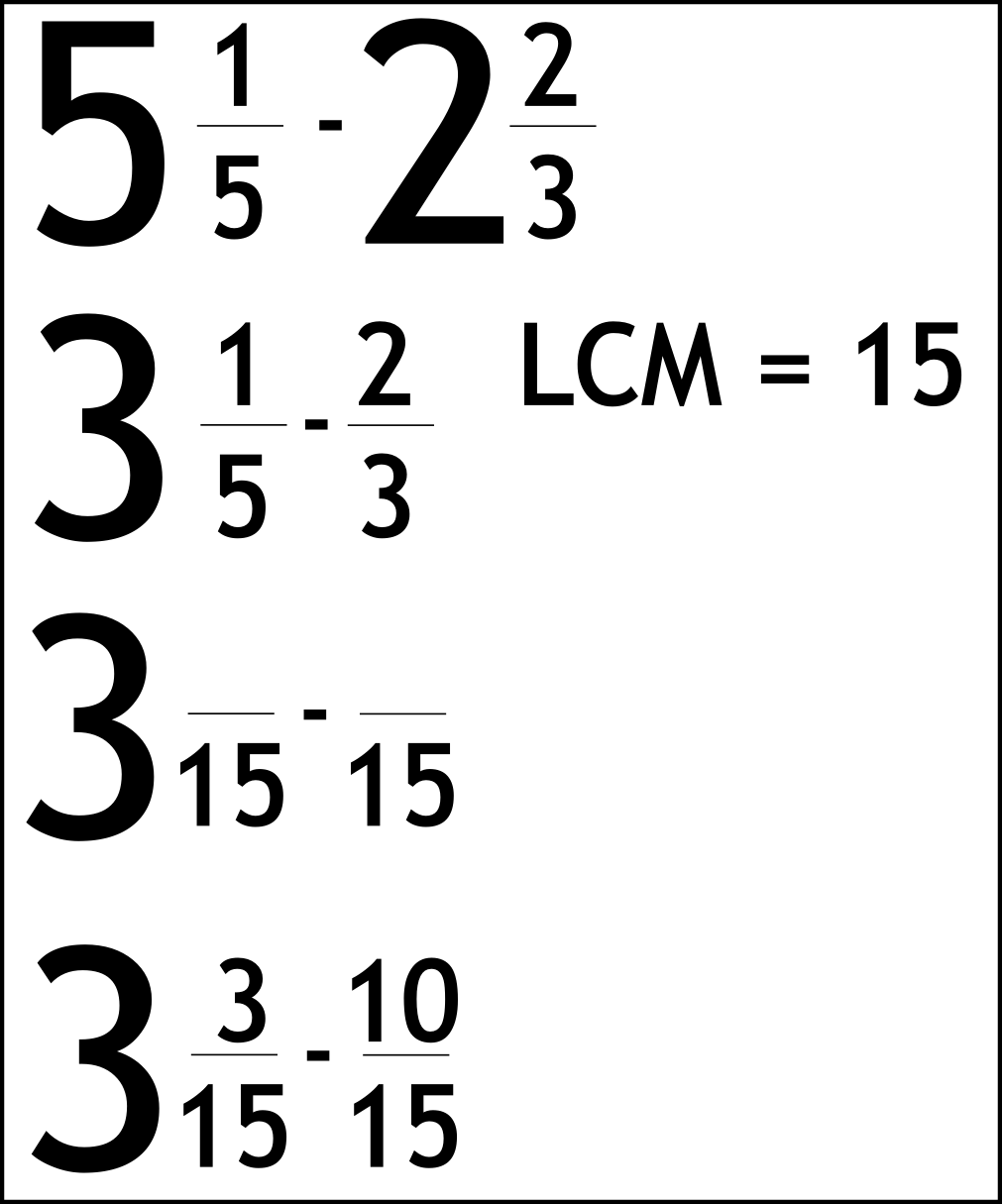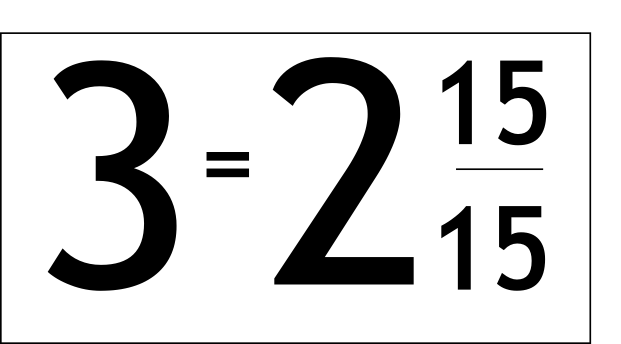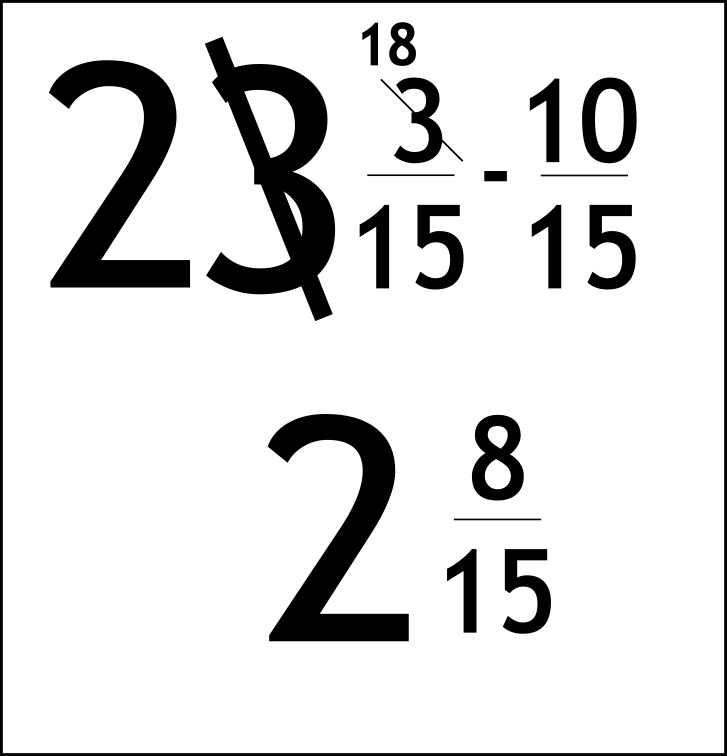The Singing Hedgehog Guide to:
Subtracting Fractions 2

Subtracting with mixed numbers is normally just the same as adding.

The only problem comes when the second fraction is smaller.

Here is an example of this sort of take away sum.Write out the question

Subtract the whole numbers
Put down the LCM

Do the "Not thinking very much at all" line

Work out what has happened to the bottoms

Do the same to the top as we did to the bottom

Write in the top values

Now we're in trouble!

All we do is the same as for a normal take away question and borrow.

We steal a whole one from the three but because we are doing fifteenths
that whole one becomes 15 fifteenths:Now we can complete the question:Borrow from the 3